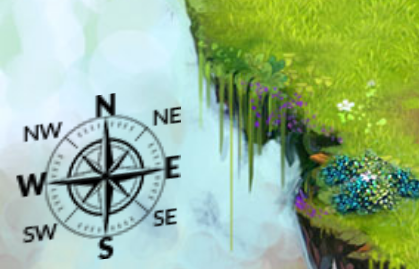## My CodeInGame Adventures - Power of Thor - Episode 1

My first CodeInGame experience was running Thor across a grassy field. We had quite the adventure, Thor and I. If it were not for me, he would have died. I'd say he owes me one!

Instructions from CodeInGame :

Thor moves on a map which is 40 wide by 18 high. Note that the coordinates (X and Y) start at the top left! This means the most top left cell has the coordinates "X=0,Y=0" and the most bottom right one has the coordinates "X=39,Y=17".

Once the program starts you are given:
the variable lightX: the X position of the light of power that Thor must reach.
the variable lightY: the Y position of the light of power that Thor must reach.
the variable initialTX: the starting X position of Thor.

the variable initialTY: the starting Y position of Thor.At the end of the game turn, you must output the direction in which you want Thor to go among:

N (North)
NE (North-East)
E (East)
SE (South-East)
S (South)
SW (South-West)
W (West)
NW (North-West)

Each movement makes Thor move by 1 cell in the chosen direction.

Here's the code I submitted:

import sys
import math

# light_x: the X position of the light of power
# light_y: the Y position of the light of power
# initial_tx: Thor's starting X position
# initial_ty: Thor's starting Y position
light_x, light_y, initial_tx, initial_ty = [int(i) for i in input().split()]
current_x = initial_tx
current_y = initial_ty

# game loop
while True:
remaining_turns = int(input())  # The remaining amount of turns Thor can move.

first = ""
second = ""
final = ""

EAST = (light_x - current_x)
WEST = (current_x - light_x)
NORTH = (current_y - light_y)
SOUTH = (light_y - current_y)

if NORTH > SOUTH:
first = "N"
current_y -=1
if ( EAST > NORTH or WEST > NORTH ):
first = ""
current_y +=1
elif NORTH < SOUTH:
first = "S"
current_y +=1
if ( EAST > SOUTH or WEST > SOUTH ):
first = ""
current_y -=1

if EAST > WEST:
second = "E"
current_x +=1
if ( NORTH > EAST or SOUTH > EAST ):
second = ""
current_x -=1
elif EAST < WEST:
second = "W"
current_x -=1
if ( NORTH > WEST or SOUTH > WEST ):
second = ""
current_x +=1

final = first + second
print(final)

#print("Debug messages...", file=sys.stderr)
# Write an action using print
# To debug: print("Debug messages...", file=sys.stderr)
# A single line providing the move to be made: N NE E SE S SW W or NW

Drop me a line if you wanna talk programming.

~ Friar Greg

By: Greg Denyes
Posted: October 14, 2017, 8:35 pm